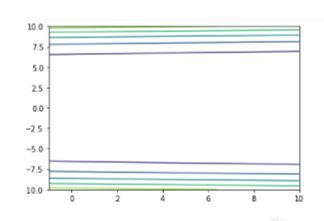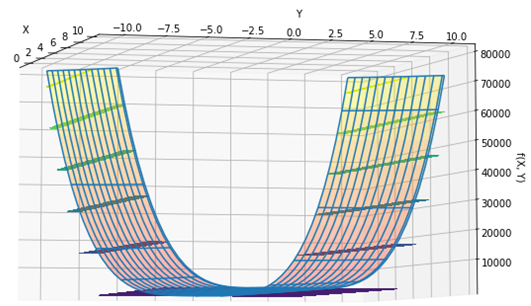top of page
Search

# DIXON-PRICE FUNCTIONMathematical DefinitionInput Domain

The function is usually evaluated on the hypercube xi ∈ [-10, 10], for all i = 1, …, d.

Global Minima

The function has global minimumDescription and Features

• The function is continuous.

• The function is scalable.

• The function is unimodal.

• The function is differentiable.

• The function is non-separable.

• The function is defined on d-dimensional space.

Python Implementation

```% Please forward any comments or bug reports in chat
Copyright 2021. INDUSMIC PRIVATE LIMITED.THERE IS NO WARRANTY, EXPRESS OR IMPLIED. WE DO NOT ASSUME ANY LIABILITY FOR THE USE OF THIS PROGRAM. If software is modified to produce derivative works, such modified software should be clearly marked. Additionally, user can redistribute it and/or modify it under the terms of the GNU General Public License. This program is distributed in the hope that it will be useful, but WITHOUT ANY WARRANTY. See the GNU General Public License for more details.
% for any support connect with us on help.indusmic@gmail.com
% Author: Ayushi Manish Shukla

from matplotlib import pyplot as plt
from mpl_toolkits import mplot3d
import numpy as np
import matplotlib.pyplot as plt
from matplotlib import cm
def f(x1, x2): return ((x1-1)**2) + (2*(2*x2**2-x1)**2)
x1 = np.linspace(-1, 10)
x2 = np.linspace(-10, 10)
X1, X2 = np.meshgrid(x1, x2)
F = f(x1,x2)
plt.contour(X1, X2, f(X1,X2))
def plotter(E,A):
fig = plt.figure(figsize=[12,8])
ax = plt.axes(projection='3d')
ax.plot_surface(X1, X2, f(X1, X2), cmap='autumn', alpha=0.3)
ax.plot_wireframe(X1,X2,f(X1,X2),rcount=15,ccount=15)
ax.view_init(elev=E, azim=A)
ax.set_xlabel('X')
ax.set_ylabel('Y')
ax.set_zlabel('f(X, Y)')
ax.contourf(x1, x2, f(X1, X2))
print("solution 2")
plotter(45,45)
from ipywidgets import interactive
iplot = interactive(plotter, E = (-90 , 90 ,5),
A = (-90 , 90 ,5))
iplot

```

References:

 Jamil, Momin, and Xin-She Yang. "A literature survey of benchmark functions for global optimization problems." International Journal of Mathematical Modelling and Numerical Optimization 4.2 (2013): 150-194.